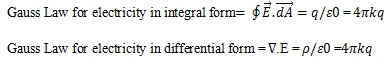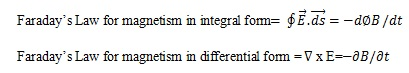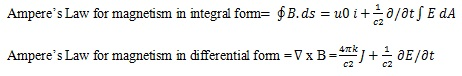# Maxwell’s Equations: Gauss’ Law, Faraday’s Law, and Ampere’s Law

The Maxwell’s equations were published by the scientist “James Clerk Maxwell” in the year 1860. These equations tell how charged atoms or elements provide electric force as well as a magnetic force for each unit charge. The energy for each unit charge is termed as the field. The elements could be motionless otherwise moving. The equations of Maxwell explain how magnetic fields can be formed by electric currents as well as charges, and finally, they explain how an electric field can produce a magnetic field, etc. The primary equation permits you to determine the electric field formed with a charge. The next equation permits you to determine the magnetic field, and the remaining two will explain how fields flow around their supplies. This article discusses Maxwell theory or Maxwell’s law. This article discusses an overview of Maxwell electromagnetic theory.

## What are Maxwell’s Equations?

The Maxwell Equation derivation is collected by four equations, where each equation explains one fact correspondingly. All these equations are not invented by Maxwell; however, he combined the four equations which are made by Faraday, Gauss, and Ampere. Although Maxwell included one part of information into the fourth equation namely Ampere’s law, that makes the equation complete.

• The first law is Gauss law intended for static electric fields
• The second law is also Gauss law intended for static magnetic fields
• The third law is Faraday’s law that tells the change of magnetic field will produce an electric field.
• The fourth law is Ampere Maxwell’s law that tells the change of electric field will produce a magnetic field.

The two equations of 3 & 4 can describe an electromagnetic wave that can spread on its own. The grouping of these equations tells that a magnetic field change can produce an electric field change, and then this will produce an additional magnetic field change. Therefore this series continues as well as an electromagnetic signal is ready as well as spreads throughout the space.

## Maxwell’s Four Equations

Maxwell’s four equations explain the two fields occurring from the supplies of electric as well as current. The fields are namely electric as well as magnetic, and how they vary within time. The four Maxwell’s equations include the following.

• First Law: Gauss’ Law for Electricity
• Second Law: Gauss’ Law for Magnetism
• Third Law: Faraday’s Law of Induction
• Fourth Law: Ampere’s Law

The above four Maxwell’s equations are Gauss for electricity, Gauss for magnetism, Faraday’s law for induction. Ampere’s law is written in different ways like Maxwell equations in integral form, and Maxwell equations in a differential form which is discussed below.

### Maxwell Equation Symbols

The symbols used in Maxwell’s equation include the following

• E denotes electrical field
• M denotes magnetic filed
• D denotes electric displacement
• H denotes magnetic field strength
• Ρ denotes charge density
• i denotes electric current
• ε0 denotes permittivity
• J denotes current density
• μ0 denotes permeability
• c denotes the speed of light
• M denotes Magnetization
• P denotes Polarization

### First Law: Gauss’ Law for Electricity

The first Maxwell’s law is Gauss law which is used for electricity. The Gauss law defines that the electric flux from any closed surface will be proportional toward the whole charge enclosed in the surface.

The Gauss’ law integral form discovers application during electric fields calculation in the region of charged objects. By applying this law to a point charge in the electric field, one can demonstrate that it is dependable with Coulomb’s law.

Although the primary region of the electric field provides a measure of the net charge included, the electric field deviation offers a measure of the compactness of sources, and also includes implication used for the protection of charge.### Second Law: Gauss’ Law for Magnetism

The second Maxwell’s law is Gauss law which is used for magnetism. The Gauss law states that the deviation of the magnetic field is equal to zero. This law applies to the magnetic flux through a closed surface. In this case, the area vector points out from the surface.

The magnetic field because of materials will be generated through a pattern named as a dipole. These poles are best signified by loops of current however be similar to positive as well as negative magnetic charges invisibly bounce together. In conditions of field lines, this law states that magnetic field lines neither start nor finish but create loops otherwise expand to infinity & reverse. In other terms, any magnetic field line that goes through a given level has to exit that volume somewhere.

This law can be written in two forms namely integral form as well as differential form. These two forms are equal because of the divergence theorem.### Third Law: Faraday’s Law of Induction

The third Maxwell’s law is Faraday’s law which is used for induction. The Faraday law states that how a time changing magnetic field will create an electric field. In integral form, it defines that the effort for every unit charge is necessary to move a charge in the region of a closed loop which equals the rate of reduction of the magnetic flux during the enclosed surface.

Similar to the magnetic field, the energetically induced electric field includes closed field lines, if not placed on by a static electric field. This electromagnetic induction feature is the working principle behind several electric generators: for instance, a magnet with a rotating bar creates a magnetic field change, which in turn produces an electric field in a near wire.### Fourth Law: Ampere’s Law

The fourth of Maxwell’s law is Ampere’s law. The Ampere’s law states that the generation of magnetic fields can be done in two methods namely with electric current as well as with changing electric fields. In integral type, the induced magnetic field in the region of any closed loop will be proportional toward the electric current and displacement current throughout the enclosed surface.

The Maxwell’s amperes law will make the set of the equations accurately reliable for non-static fields without altering the Ampere as well as Gauss laws for fixed fields. But as a result, it expects that a change of the magnetic field will induce an electric field. Thus, these mathematical equations will allow self-sufficient electromagnetic wave for moving through empty space. The electromagnetic waves speed can be measured and that could be expected from the currents as well as charges experiments match the light’s speed, and this is one type of electromagnetic radiation.∇ x B = J/ε0c2+ 1/c2 ∂E/∂t

Thus, this is all about Maxwell’s equations. From the above equations, finally, we can conclude that these equations include four laws that are related to the electric (E) as well as magnetic (B) field are discussed above. Maxwell’s equations may be written in the form of equivalent integral as well as differential. Here is a question for you, what are the applications of Maxwell’s Equations?